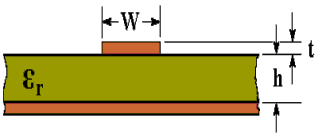# Microstrip Transmission Line Characteristic Impedance Calculator

The characteristic impedance (Zo) of a microstrip transmission line can be calculated using the active calculator or the formulas at the bottom of the page.Enter the εr of the PCB: Enter the width of the track: mm Enter the thickness of the track: mm Effective Dielectric Constant (εeff): Enter the thickness of the dielectric: mm Characteristic Impedance (Zo): Ohms

This calculator uses Visual Basic and will work in Internet Explorer and other browsers with Visual Basic plug-ins. For more information see About our calculators

The following formulas are from an article by Rick Hartley page 27 http://www.jlab.org/accel/eecad/pdf/050rfdesign.pdf

The formulas give results within 3% of the results obtained in http://www.polarinstruments.com/support/cits/IPC1999.pdf

More calculators for transmission line can be found at http://www.circuitsage.com/tline.htmlWhere εr is the Relative Dielectric Constant

W is the Width of track

t is the Thickness of track (1 oz/ft2 = 0.035 mm)

h is the Thickness of dielectric

This calculator is provided free by Chemandy Electronics in order to promote the FLEXI-BOX